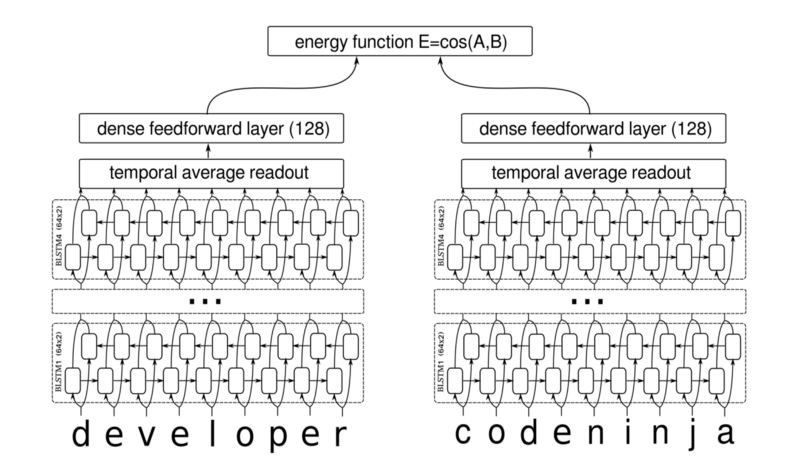Blogs
November 21, 2017

# Semantic Similarity - Next Steps to Topic Modeling

Semantic similarity is a subfield in NLP which deals with classifying two input text as similar or not. Traditional methods have used various metrics like the hamming distance, Levenshtein Distance, etc, to find similarity between words. Metrics like topological similarity can be used to estimate similarity between sentences.

e.g. These statements are not similar

These statements have high similarity

One of the observation from above examples is two similar statements will not necessarily have the same number of words or exact set of words. Plus the task of similarity can be viewed as multi class classification or a continuous function as opposed to binary classification, as two statements might have a varying degree of similarity.

#### Use in Chatbot

In our chatbot, we use semantic similarity for question and answering. In our real estate chatbot, we have a knowledge base section whose function is to return the appropriate answer from the knowledge base given an input user question. To construct knowledge base we first scrap data from various real estate website and clean it removing all HTML code. This data is then passed to question generator to generate question and answer pairs. We use the system developed by Michael Heilman and Noah A. Smith “Question Generation via Overgenerating Transformations and Ranking” for generation of q&a pair. Whenever the user enters a question we use semantic similarity to find the semantically most similar question and use its answer to craft the response.

#### Techniques for Semantic similarity

As mentioned above topological similarity is one of the methods that can be used to do this. It works by creating ontologies that define the relationship between terms and concepts. Constructing an ontology can be a difficult task and useful if doing large scale work like in medical domain. We also need an approach which is robust to the addition of negative words or any other kind of words that might change the meaning of the sentence. In our application, we have explored deep learning models for this purpose.

#### Siamese LSTM network

This model takes word vector and character embedding as input. Word vectors are very useful in this problem as word vector work by capturing word meaning. Hence words like ‘clever’ and ‘wise’ are close in the vector space. When these word vectors are passed through a model which works on sequence they can capture similarity based on context. We use the recurrent neural network with LSTM units for this. In this Siamese LSTM network, we have two LSTM network that accepts two statements and computes representation at the end. We can then apply metrics like cosine similarity to find similarity score.
The input can be character embedding

or word + character embedding.

#### Semantic similarity using convolution neural network

This model uses convolutional neural network for semantic similarity.
Quote from the original paper.

To summarize, our model (shown in Figure 1) consists of two main components:
A sentence model for converting a sentence into a representation for similarity measurement; we use a convolutional neural network architecture with multiple types of convolution and pooling in order to capture different granularities of information in the inputs.
A similarity measurement layer using multiple similarity measurements, which compare local regions of the sentence representations from the sentence model.

The convolution neural network computes hierarchical structure over input much like recursive neural network does. This kind of hierarchical structure captures and uses various feature of sentence like the dependency tree.

Model overview Hua He, et al.,”Multi-Perspective Sentence Similarity Modeling with Convolutional Neural Networks” source: http://aclweb.org/anthology/D/D15/D15-1181.pdf